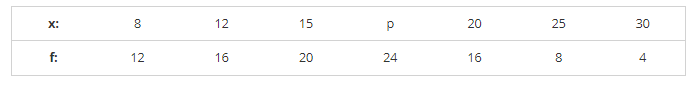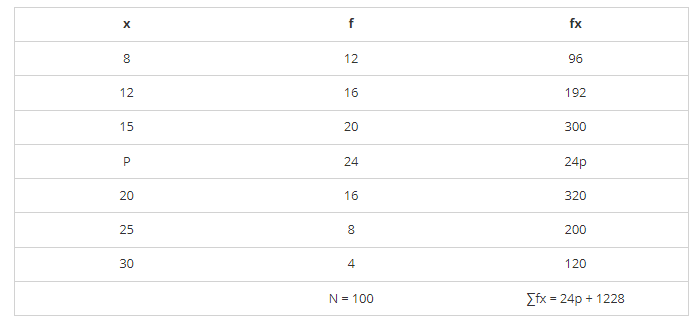# Find the value of p for the following distribution whose mean is 16.6.

Question:

Find the value of p for the following distribution whose mean is 16.6.Solution:It is given that,

Mean = 16.6

$\Rightarrow \frac{\sum \mathrm{fx}}{\mathrm{N}}=16.6$

$\Rightarrow \frac{24 \mathrm{p}+1228}{100}=16.6$

⇒ 24p + 1228 = 1660

⇒ 24p = 1660 − 1228

⇒ 24p = 432

⇒ p = 432/24 = 18

⇒ p = 18

∴ p = 18.